## Compute the percentage of error to the nearest tenth in the student’s calculation if the actual specific heat value for aluminum is 0.9J:g*C

Question

Compute the percentage of error to the nearest tenth in the student’s calculation if the actual specific heat value for aluminum is 0.9J:g*C.

in progress 0
3 weeks 2021-09-02T20:25:20+00:00 1 Answers 0 views 0

1. Complete Question:

1. A block of aluminum with a mass of 140 g is cooled from 98.4°C to 62.2°C with a release of 4817 J of heat. From these data, calculate the specific heat of aluminum.

a. Compute the percentage of error to the nearest tenth in the student’s calculation if the actual specific heat value for aluminum is 0.9 J/g°C

Percent error = 55.56 %

Explanation:

Given the following data;

Mass = 140 grams

Initial temperature = 62.2°C

Final temperature = 98.4°C

Quantity of heat = 4817 Joules.

To find the specific heat capacity;

Heat capacity is given by the formula;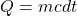Where;

• Q represents the heat capacity or quantity of heat.
• m represents the mass of an object.
• c represents the specific heat capacity of water.
• dt represents the change in temperature.

dt = T2- T1

dt = 98.4 – 62.2

dt = 36.2°C

Making c the subject of formula, we have;Substituting into the equation, we have;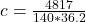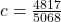Specific heat capacity, = 0.95 J/g°C

b. To find the percentage error;

Given the following data;

Actual specific heat capacity = 0.9 J/g°C

Experimental specific heat capacity = 0.95 J/g°C

Percent error can be defined as a measure of the extent to which an experimental value differs from the theoretical value.

Mathematically, it is given by this expression;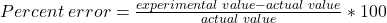Substituting into the formula, we have;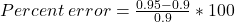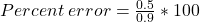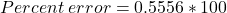Percent error = 55.56 %